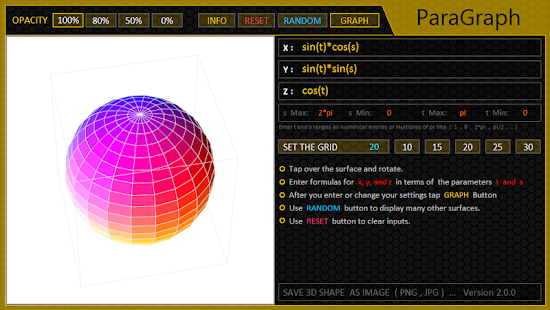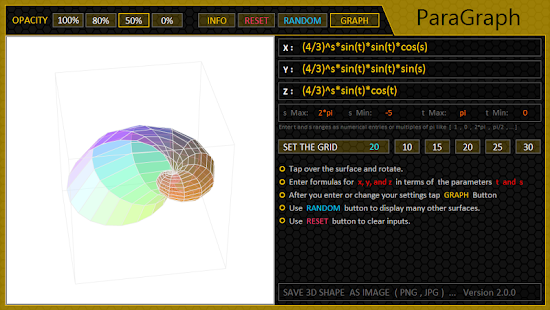# Grapher Pro 3D v2.2.3 APK is Here ! [Latest]## Grapher Pro 3D

Parametric Grapher. Draw 2D and 3D Advanced Parametric or Simple graph.

3D Parametric Graph :
Enter formulas for the three coordinates in terms of the parameters t and s.

2D Simple Graph :
Enter formula just for the one coordinate in terms of the parameters t or s.
After you enter formulas tap the GRAPH …

You can change the grid, opacity of a surface displayed, and rotate a surface.
Exmp: 2*t^2+sin(s)-t/2
* : Multiplication
/ : Division
^ : Exponentiation
– : Subtraction
Trigonometric & Inverse Trigonometric Functions:
sin( ) , cos( ) , tan( ) , asin( ) , acos( ) , atan( )
Exmp : tan(pi*t/3)+acos(s)
Use pi for the constant pi ; 3,14…
Use ln( ) for the natural logarithm, e for the natural base.
Exmp : e^(2-s)*ln(t^2+1)
sqrt( ) for the square root abs( ) for the absolute value
max( , ) for the maximim of two entries
min( , ) for the minimum of two entriesfloor( ) , ceil( ) , round( ) for the previous, next, and the nearest integer …

What’s New

••